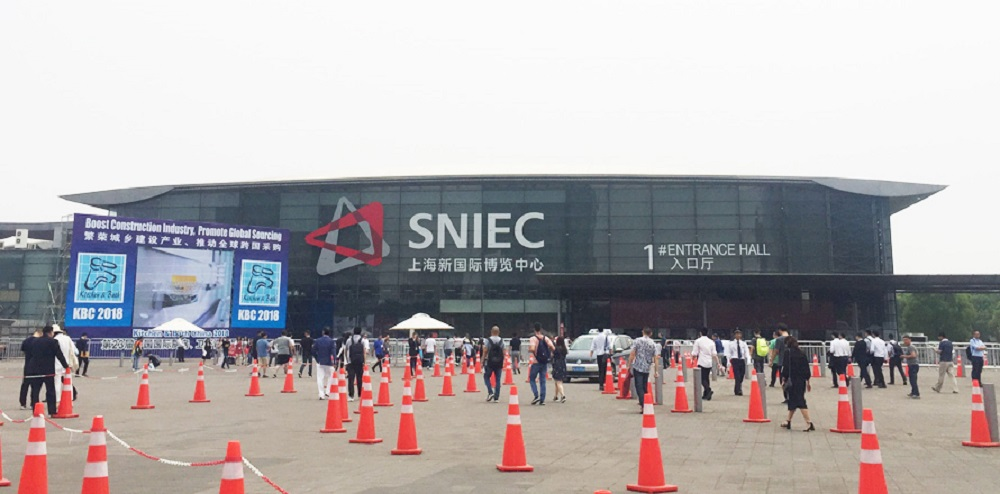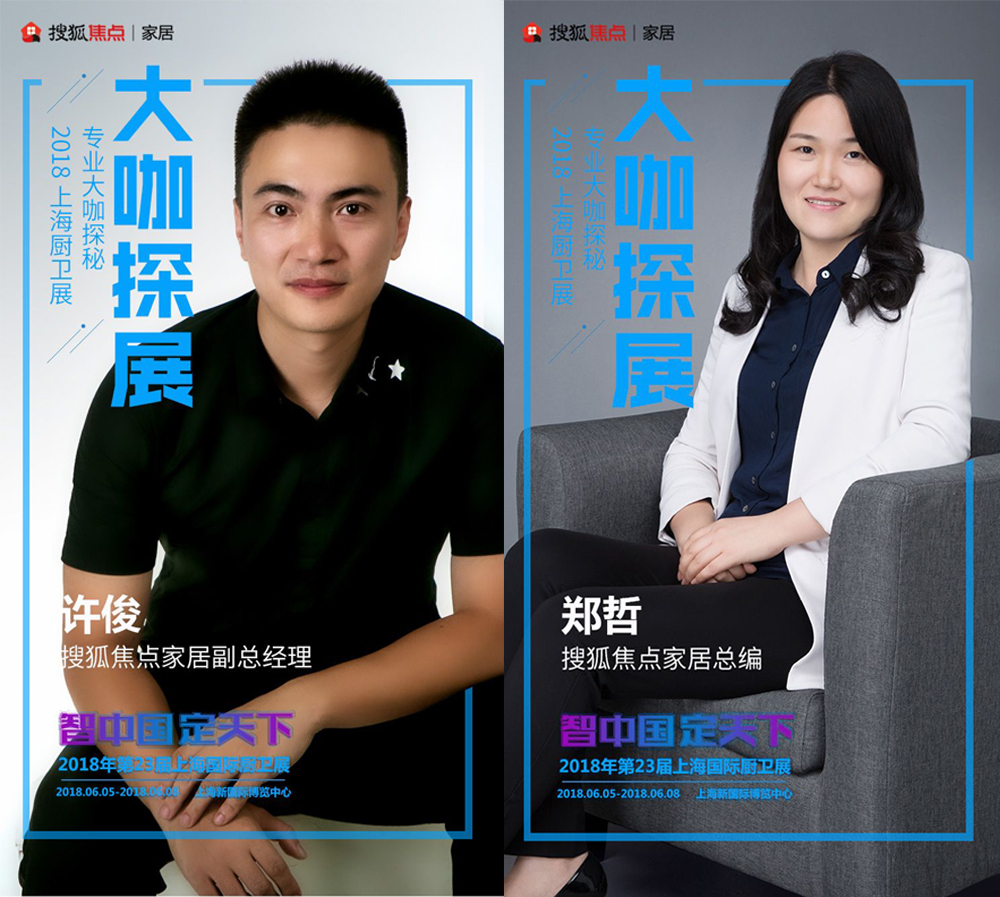|

# 大咖探展:卫浴行业明星企业,都在做什么?

【导语】2018年上海厨卫展，25万平方米，全新的规模；17个展馆，全新的机遇；全球1000多家参展企业，全新的产品；在这一届上海厨卫展上，搜狐焦点家居特别活动——“大咖探展”，带着大家一起走最少的路，看最亮的点!2018年6月5日至6日，第23届中国国际厨房、卫浴设施展览在上海新国际博览中心正式拉开了帷幕，作为行业内一大盛事，今年吸引了海内外多家展商参与这个“厨卫奥斯卡”。展会期间，搜狐焦点家居特别策划活动“大咖探展”的两位 “团长”分别进行了一趟直播逛展之旅， 一览厨卫行业大牌们的吸睛展位和点睛单品，并以“智中国 定天下”为主题，与九牧副总裁严桢先生、安华卫浴事业部总经理温尊华先生、恒洁市场副总裁阮伟华女士、东鹏洁具杨立鑫先生、欧路莎董事长林华友先生、浪鲸卫浴品牌中心总监林学舟先生六位行业“大佬”进行了深度对话，聊聊中国卫浴行业的“那些年“、“那些事”以及“那些人儿”。直播探展的第一站:九牧展馆

此次展会，九牧以超大的展区规模、前瞻的智能科技闪耀登场，展出了一款高端匠心之作——阳厨卫一体化套间“M6名匠“，这一款新品从材质和色彩皆令人眼前一亮，充分地表现出九牧对工匠精神的极致追求。

直播期间，九牧副总裁严桢先生热情洋溢地简单介绍了新产品，并分享了许多睿智的观点，他说：”每个企业先要以产品为重，致力于完善售后服务，同时企业要有社会责任感，切切实实地去做公益，也要坚持长期不断地做下去！“

（长按图片观看直播视频）

直播探展的第二站: 安华卫浴展馆

安华卫浴此次的展位号为W2B15，参展产品包括了坐便器、浴室柜、定制卫浴空间等一系列新整体卫浴新产品，而其中的两款新品——智能马桶i10、i11最为亮眼，吸引了众多参展人的目光。

直播期间，安华卫浴事业部总经理温尊华先生跟探展“团长”郑哲女士边看展馆边聊，并且表示了对i10、i11这两款新品的满满信心。在轻松愉快的直播聊天中，他表示：“这次是我们新形象升级后的首次展会，安华卫浴在今年年初发布了全新的品牌VI，品牌诉求是‘轻奢、时尚、简约、高性价比‘，在产品各方面都进行革新和升级。“

（长按图片观看直播视频）

直播探展的第三站: 恒洁卫浴展馆

“节水“和”智能“，一向是是恒洁卫浴科技的关键词，”营造更舒适、贴心的智能卫浴体验“，一向是安华卫浴的主修课。此次展会中，恒洁以简奢系列和摩登都市系列产品亮相，展现了不一般的简奢、摩登卫浴风格。

直播期间，作为卫浴界“女强人“般的存在——恒洁市场副总裁阮伟华女士与探展“团长”许俊先生展开了深度对话，她表示： “恒洁一直重视产品喝核心技术的研发，创新要渗透到企业的方方面面。在将来的发展中，恒洁有信心做得更好，打造更多质感好产品。“

（长按图片观看直播视频）

直播探展的第四站: 东鹏洁具展馆

东鹏洁具此次以“漂亮房子，东鹏造”的主题参展，从展馆的外观设计到内部布局，从产品的陈列展出到互动推广，从品牌的外在形象到内在气质，都会透露着一股时尚新风，而此次展出的新生代超光盾系列智能马桶W8111、北欧风宜家系列浴室柜均体现了这一特点。

直播期间，东鹏杨立鑫先生热情地接待了探展“团长“郑哲女士，并带领探展”团长“逛起了东鹏的展馆。对话过程中，杨立鑫先生的一番话引得了”团长“的赞同，他说：“近几年，东鹏通过自身的技术研发、与国外大牌合作，在卫浴整装板块也是越做越好。同时，企业的发展要关注到消费者的需求，做好品牌形象管理。”

（长按图片观看直播视频）

直播探展的第五站: 欧路莎卫浴展馆

此次展会，欧路莎卫浴展馆整体呈现出高端、简约的风格，外围采用三角形、平行四边形、梯形等各种几何图形组合，营造出立体、开放的展馆空间氛围，无论哪个角度，均能窥见展馆内的场景。其中，欧路莎今年最新研制的概念款"外星"智能马桶IT-809重磅现身，其球形外观设计吸引了众多关注。

直播期间，欧路莎董事长林华友先生首先介绍了此次的新品，他表示：“产品设计要提高消费者的使用体验，能关注到消费者的多种需求，这是欧路莎与其他品牌的最大区分点。中国卫浴行业同质化严重，创新是欧路莎的出路，要做原创的绿色家居产品。”

（长按图片观看直播视频）

直播探展的第六站:浪鲸卫浴展馆

浪鲸卫浴，作为有个性的卫浴品牌，从精工细致的浴缸，到意式风情浓郁的浴室柜系列产品，既传承了匠心精神，也以创新技术完成了对工业艺术的追求。

直播期间，浪鲸卫浴品牌中心总监林学舟先生是位健谈的人，首先他跟探展“团长“郑哲女士就“性感的家居”话题展开谈论，他表示：“我们今年展出的产品多是意式风格，一直致力于做高端的定制家居，做一个散发魅力的”性感 “品牌，而浪鲸也会让消费者认同这个别具一格的品牌概念。“

（长按图片观看直播视频）

我相信，通过这次的探展活动，大家会对上海厨卫展能了解多一些，对于卫浴行业也慢慢能看出些“门道”来!欲知更多2018上海厨卫展的详细信息，敬请点击下图，浏览搜狐焦点家居2018上海厨卫展专题。

(长按图片观看搜狐焦点上海厨卫展专题)

`声明：本文由入驻焦点开放平台的作者撰写，除焦点官方账号外，观点仅代表作者本人，不代表焦点立场错误信息举报电话： 400-099-0099，邮箱：jubao@vip.sohu.com，或点此进行意见反馈，或点此进行举报投诉。`A B C D E F G H J K L M N P Q R S T W X Y Z
A - B - C - D - E
• A
• 鞍山
• 安庆
• 安阳
• 安顺
• 安康
• 澳门
• B
• 北京
• 保定
• 包头
• 巴彦淖尔
• 本溪
• 蚌埠
• 亳州
• 滨州
• 北海
• 百色
• 巴中
• 毕节
• 保山
• 宝鸡
• 白银
• 巴州
• C
• 承德
• 沧州
• 长治
• 赤峰
• 朝阳
• 长春
• 常州
• 滁州
• 池州
• 长沙
• 常德
• 郴州
• 潮州
• 崇左
• 重庆
• 成都
• 楚雄
• 昌都
• 慈溪
• 常熟
• D
• 大同
• 大连
• 丹东
• 大庆
• 东营
• 德州
• 东莞
• 德阳
• 达州
• 大理
• 德宏
• 定西
• 儋州
• 东平
• E
• 鄂尔多斯
• 鄂州
• 恩施
F - G - H - I - J
• F
• 抚顺
• 阜新
• 阜阳
• 福州
• 抚州
• 佛山
• 防城港
• G
• 赣州
• 广州
• 桂林
• 贵港
• 广元
• 广安
• 贵阳
• 固原
• H
• 邯郸
• 衡水
• 呼和浩特
• 呼伦贝尔
• 葫芦岛
• 哈尔滨
• 黑河
• 淮安
• 杭州
• 湖州
• 合肥
• 淮南
• 淮北
• 黄山
• 菏泽
• 鹤壁
• 黄石
• 黄冈
• 衡阳
• 怀化
• 惠州
• 河源
• 贺州
• 河池
• 海口
• 红河
• 汉中
• 海东
• 怀来
• I
• J
• 晋中
• 锦州
• 吉林
• 鸡西
• 佳木斯
• 嘉兴
• 金华
• 景德镇
• 九江
• 吉安
• 济南
• 济宁
• 焦作
• 荆门
• 荆州
• 江门
• 揭阳
• 金昌
• 酒泉
• 嘉峪关
K - L - M - N - P
• K
• 开封
• 昆明
• 昆山
• L
• 廊坊
• 临汾
• 辽阳
• 连云港
• 丽水
• 六安
• 龙岩
• 莱芜
• 临沂
• 聊城
• 洛阳
• 漯河
• 娄底
• 柳州
• 来宾
• 泸州
• 乐山
• 六盘水
• 丽江
• 临沧
• 拉萨
• 林芝
• 兰州
• 陇南
• M
• 牡丹江
• 马鞍山
• 茂名
• 梅州
• 绵阳
• 眉山
• N
• 南京
• 南通
• 宁波
• 南平
• 宁德
• 南昌
• 南阳
• 南宁
• 内江
• 南充
• P
• 盘锦
• 莆田
• 平顶山
• 濮阳
• 攀枝花
• 普洱
• 平凉
Q - R - S - T - W
• Q
• 秦皇岛
• 齐齐哈尔
• 衢州
• 泉州
• 青岛
• 清远
• 钦州
• 黔南
• 曲靖
• 庆阳
• R
• 日照
• 日喀则
• S
• 石家庄
• 沈阳
• 双鸭山
• 绥化
• 上海
• 苏州
• 宿迁
• 绍兴
• 宿州
• 三明
• 上饶
• 三门峡
• 商丘
• 十堰
• 随州
• 邵阳
• 韶关
• 深圳
• 汕头
• 汕尾
• 三亚
• 三沙
• 遂宁
• 山南
• 商洛
• 石嘴山
• T
• 天津
• 唐山
• 太原
• 通辽
• 铁岭
• 泰州
• 台州
• 铜陵
• 泰安
• 铜仁
• 铜川
• 天水
• 天门
• W
• 乌海
• 乌兰察布
• 无锡
• 温州
• 芜湖
• 潍坊
• 威海
• 武汉
• 梧州
• 渭南
• 武威
• 吴忠
• 乌鲁木齐
X - Y - Z
• X
• 邢台
• 徐州
• 宣城
• 厦门
• 新乡
• 许昌
• 信阳
• 襄阳
• 孝感
• 咸宁
• 湘潭
• 湘西
• 西双版纳
• 西安
• 咸阳
• 西宁
• 仙桃
• 西昌
• Y
• 运城
• 营口
• 盐城
• 扬州
• 鹰潭
• 宜春
• 烟台
• 宜昌
• 岳阳
• 益阳
• 永州
• 阳江
• 云浮
• 玉林
• 宜宾
• 雅安
• 玉溪
• 延安
• 榆林
• 银川
• Z
• 张家口
• 镇江
• 舟山
• 漳州
• 淄博
• 枣庄
• 郑州
• 周口
• 驻马店
• 株洲
• 张家界
• 珠海
• 湛江
• 肇庆
• 中山
• 自贡
• 资阳
• 遵义
• 昭通
• 张掖
• 中卫

1室1厅1厨1卫1阳台

1
2
3
4
5

0
1
2

1

1

0
1
2
3报名成功，资料已提交审核A B C D E F G H J K L M N P Q R S T W X Y Z
A - B - C - D - E
• A
• 鞍山
• 安庆
• 安阳
• 安顺
• 安康
• 澳门
• B
• 北京
• 保定
• 包头
• 巴彦淖尔
• 本溪
• 蚌埠
• 亳州
• 滨州
• 北海
• 百色
• 巴中
• 毕节
• 保山
• 宝鸡
• 白银
• 巴州
• C
• 承德
• 沧州
• 长治
• 赤峰
• 朝阳
• 长春
• 常州
• 滁州
• 池州
• 长沙
• 常德
• 郴州
• 潮州
• 崇左
• 重庆
• 成都
• 楚雄
• 昌都
• 慈溪
• 常熟
• D
• 大同
• 大连
• 丹东
• 大庆
• 东营
• 德州
• 东莞
• 德阳
• 达州
• 大理
• 德宏
• 定西
• 儋州
• 东平
• E
• 鄂尔多斯
• 鄂州
• 恩施
F - G - H - I - J
• F
• 抚顺
• 阜新
• 阜阳
• 福州
• 抚州
• 佛山
• 防城港
• G
• 赣州
• 广州
• 桂林
• 贵港
• 广元
• 广安
• 贵阳
• 固原
• H
• 邯郸
• 衡水
• 呼和浩特
• 呼伦贝尔
• 葫芦岛
• 哈尔滨
• 黑河
• 淮安
• 杭州
• 湖州
• 合肥
• 淮南
• 淮北
• 黄山
• 菏泽
• 鹤壁
• 黄石
• 黄冈
• 衡阳
• 怀化
• 惠州
• 河源
• 贺州
• 河池
• 海口
• 红河
• 汉中
• 海东
• 怀来
• I
• J
• 晋中
• 锦州
• 吉林
• 鸡西
• 佳木斯
• 嘉兴
• 金华
• 景德镇
• 九江
• 吉安
• 济南
• 济宁
• 焦作
• 荆门
• 荆州
• 江门
• 揭阳
• 金昌
• 酒泉
• 嘉峪关
K - L - M - N - P
• K
• 开封
• 昆明
• 昆山
• L
• 廊坊
• 临汾
• 辽阳
• 连云港
• 丽水
• 六安
• 龙岩
• 莱芜
• 临沂
• 聊城
• 洛阳
• 漯河
• 娄底
• 柳州
• 来宾
• 泸州
• 乐山
• 六盘水
• 丽江
• 临沧
• 拉萨
• 林芝
• 兰州
• 陇南
• M
• 牡丹江
• 马鞍山
• 茂名
• 梅州
• 绵阳
• 眉山
• N
• 南京
• 南通
• 宁波
• 南平
• 宁德
• 南昌
• 南阳
• 南宁
• 内江
• 南充
• P
• 盘锦
• 莆田
• 平顶山
• 濮阳
• 攀枝花
• 普洱
• 平凉
Q - R - S - T - W
• Q
• 秦皇岛
• 齐齐哈尔
• 衢州
• 泉州
• 青岛
• 清远
• 钦州
• 黔南
• 曲靖
• 庆阳
• R
• 日照
• 日喀则
• S
• 石家庄
• 沈阳
• 双鸭山
• 绥化
• 上海
• 苏州
• 宿迁
• 绍兴
• 宿州
• 三明
• 上饶
• 三门峡
• 商丘
• 十堰
• 随州
• 邵阳
• 韶关
• 深圳
• 汕头
• 汕尾
• 三亚
• 三沙
• 遂宁
• 山南
• 商洛
• 石嘴山
• T
• 天津
• 唐山
• 太原
• 通辽
• 铁岭
• 泰州
• 台州
• 铜陵
• 泰安
• 铜仁
• 铜川
• 天水
• 天门
• W
• 乌海
• 乌兰察布
• 无锡
• 温州
• 芜湖
• 潍坊
• 威海
• 武汉
• 梧州
• 渭南
• 武威
• 吴忠
• 乌鲁木齐
X - Y - Z
• X
• 邢台
• 徐州
• 宣城
• 厦门
• 新乡
• 许昌
• 信阳
• 襄阳
• 孝感
• 咸宁
• 湘潭
• 湘西
• 西双版纳
• 西安
• 咸阳
• 西宁
• 仙桃
• 西昌
• Y
• 运城
• 营口
• 盐城
• 扬州
• 鹰潭
• 宜春
• 烟台
• 宜昌
• 岳阳
• 益阳
• 永州
• 阳江
• 云浮
• 玉林
• 宜宾
• 雅安
• 玉溪
• 延安
• 榆林
• 银川
• Z
• 张家口
• 镇江
• 舟山
• 漳州
• 淄博
• 枣庄
• 郑州
• 周口
• 驻马店
• 株洲
• 张家界
• 珠海
• 湛江
• 肇庆
• 中山
• 自贡
• 资阳
• 遵义
• 昭通
• 张掖
• 中卫• 手机• 分享
• 设计
免费设计
• 计算器
装修计算器
• 入驻
合作入驻
• 联系
联系我们
• 置顶
返回顶部